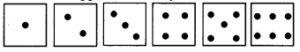# Maharashtra Board 10th Class Maths Part 1 Practice Set 5.1 Solutions Chapter 5 Probability

Balbharti Maharashtra State Board Class 10 Maths Solutions covers the Practice Set 5.1 Algebra 10th Class Maths Part 1 Answers Solutions Chapter 5 Probability.

## Practice Set 5.1 Algebra 10th Std Maths Part 1 Answers Chapter 5 Probability

Question 1.
How many possibilities are there in each of the following?
i. Vanita knows the following sites in Maharashtra. She is planning to visit one of them in her summer vacation. Ajintha, Mahabaleshwar, Lonar Sarovar, Tadoba wild life sanctuary, Amboli, Raigad, Matheran, Anandavan.
ii. Any day of a week is to be selected randomly.
iii. Select one card from the pack of 52 cards.
iv. One number from 10 to 20 is written on each card. Select one card randomly.
Solution:
i. Here, 8 sites of Maharashtra are given.
∴ There are 8 possibilities in a random experiment of visiting a site out of 8 sites in Maharashtra.

ii. There are 7 days in a week.
∴ There are 7 possibilities in a random experiment of selecting a day of the week.

iii. There are 52 cards in a pack of cards.
∴ There are 52 possibilities in a random experiment of selecting one card from the pack of 52 cards.

iv. There are 11 cards numbered from 10 to 20.
∴ There are 11 possibilities in a random experiment of selecting one card from the given set of cards.

Question 1.
In which of the following experiments possibility of expected outcome is more? (Textbook pg, no. 116)
i. Getting 1 on the upper face when a die is thrown.
ii. Getting head by tossing a coin.
Solution:
i. On a die there are 6 numbers.
∴ There are 6 possibilities of getting any one number from 1 to 6 on the upper face i.e. $$\frac { 1 }{ 6 }$$ is the possibility.

ii. There are two possibilities (H or T) on tossing a coin i.e. $$\frac { 1 }{ 2 }$$ possibility.
∴ In the second experiment, the possibility of expected outcome is more.

Question 2.
Throw a die, once. What are the different possibilities of getting dots on the upper face? (Textbook pg. no. 114)